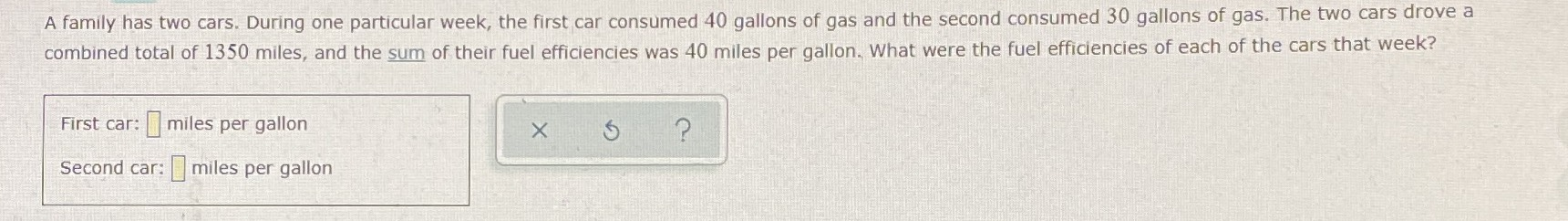### ¿Todavía tienes preguntas de matemáticas?

Pregunte a nuestros tutores expertos
Algebra
PreguntaA family has two cars. During one particular week, the first car consumed $$40$$ gallons of gas and the second consumed $$30$$ gallons of gas. The two cars drove a combined total of $$1350$$ miles, and the sum of their fuel efficiencies was $$40$$ miles per gallon. What were the fuel efficiencies of each of the cars that week?